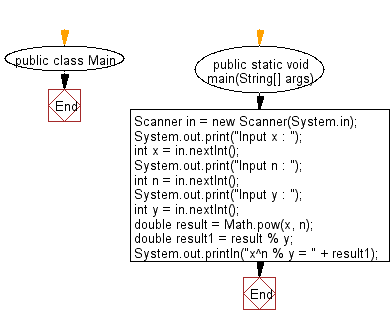# Java Exercises: Compute xn % y where x, y and n are all 32bit integers

## Java Basic: Exercise-204 with Solution

Write a Java program to compute xn % y where x, y and n are all 32bit integers.

Sample Solution:

Java Code:

``````import java.util.*;
public class Main
{
public static void main(String[] args)
{
Scanner in = new Scanner(System.in);
System.out.print("Input x : ");
int x = in.nextInt();
System.out.print("Input n : ");
int n = in.nextInt();
System.out.print("Input y : ");
int y = in.nextInt();
double result = Math.pow(x, n);
double result1 = result % y;
System.out.println("x^n % y = " + result1);
}
}
```
```

Sample Output:

```Input x :  25
Input n :  35
Input y :  45
x^n % y = 5.0
```

Flowchart:Java Code Editor:

What is the difficulty level of this exercise?

﻿

## Java: Tips of the Day

Directory Content:

Java allows you to get the names of all subdirectories and files in a folder as an array, which can then be sequentially viewed.

```import java.io.*;

public class ListContents {
public static void main(String[] args) {
File file = new File("//home//user//Documents/");
String[] files = file.list();

System.out.println("Listing contents of " + file.getPath());
for(int i=0 ; i < files.length ; i++)
{
System.out.println(files[i]);
}
}
}
```